Electronic Control Theory of Second-Order Systems: A Practical Analysis for Engineers

Abstract

This article brings control theory to life. Too often control theory is taught using block diagrams with no reference to real life circuitry. With the help of some math and a circuit simulator, it can be shown that electronic control theory has relevance in modern electronic circuit design.

Introduction

Many subjects are taught in university that solicit the response from the student: “Will this get me a job?” Control theory might well be one of those subjects with no immediate use for pages of math and block diagrams of feedback systems. However, control theory teaches the engineer how to design systems that behave themselves, how close to the boundaries of stable operation a system is, and how to get the best response from any given system. Whether the subject is mechanical, electrical, civil, aeronautical, or communications engineering, if a system is not stable, it is of no use in the real world.

To the design engineer, control theory is life itself.

There are many excellent texts written on control theory, but many of them take a generalist approach, illustrated by block diagrams. This article is written for the electronics engineer and introduces electronic control theory from the viewpoint of circuit analysis and simulation. It explains the theory behind general second-order systems but illustrates the theory with worked circuit examples. The aim is to demystify the basics of second-order systems and explain to anyone trying to learn electronic control theory that it has relevance in analog circuit design.

Second-Order Systems

The most basic second-order network is shown in Figure 1.Figure 1. A second-order network consisting of a resistor, an inductor, and a capacitor.

This has a transfer function of

The denominator of the right hand side of Equation 1 is known as the characteristic polynomial and if we equate the characteristic polynomial to zero, we get the characteristic equation. The poles of a system occur when the denominator of its transfer function equals zero. By finding the roots of the characteristic equation (the values of s that make the characteristic equation equal to zero), we can find the poles of the system and hence discover a wealth of information about how the system behaves.

The general form of a second-order system transfer function is given by

where ζ is the damping coefficient and ωn is the circuit’s natural frequency (or undamped frequency) of oscillation in radians per second.

Therefore, the general characteristic equation for a second-order system is given by:

Comparing Equation 3 with Equation 1, we can see that the circuit in Figure 1 has a natural frequency represented by:

We can also see that the resistance in the circuit plays a part in the damping coefficient of the network:

so

so

This is intuitive—if the circuit has no resistance, there is no loss in the network (no damping) so if the circuit is stimulated, it will oscillate forever. As resistance is increased, the oscillation decays more rapidly.

Figure 2 shows an RLC circuit excited with a 1 V step input with values L = 1 µH and C = 1 µF, and resistances of 0 Ω, 100 mΩ, and 500 mΩ. The circuit oscillates at a frequency of 159 kHz, as expected. The effect of increased resistance on decay is clear.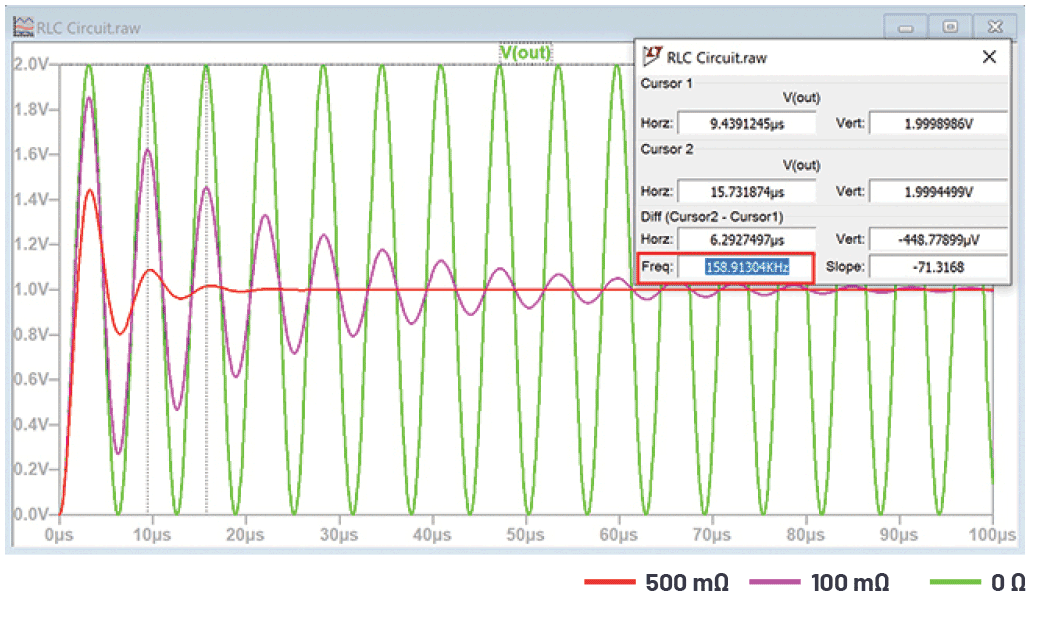Figure 2. The effect of resistance on damping the oscillation of a network.

The results of the simulation shown in Figure 2 can be shown mathematically by translating from the Laplace domain to the time domain. A unit step input in the Laplace domain is represented by

so when a second-order system is stimulated by a unit step input, the response becomes

Using partial fraction expansion, Equation 9 can be represented as

Equation 10 is represented in the Laplace domain.

In the time domain, this translates to

where

A mathematical derivation of Equation 11 with an inverse Laplace transform is shown in Appendix A.

Equation 11 tells us how the circuit in Figure 1 responds to a step input. We can see that the waveform has a sinusoidal-like nature and its amplitude is modulated by the term e–ζωnt, which decays or grows exponentially depending on whether the damping coefficient is positive or negative. As an approximation, we can see that the response consists of a sinusoidal part and a cosinusoidal part, but, for low damping coefficients, the sinusoidal part is small.

Moreover, we can see that, although the natural frequency of the circuit is ωn, the circuit does not oscillate at this frequency but rather a frequency, ωd, that is somewhat lower and determined by the damping coefficient, ζ. This frequency is known as the damped natural frequency. Nevertheless, the exponential decay is dependent on the undamped natural frequency of the circuit, ωn.

The poles of a transfer function are found by determining when the denominator of the transfer function is equal to zero, namely:

This can be solved for s using the quadratic formula:

where

a = 1

b = 2ζωn

c = ωn2

The poles of the system occur when

If the damping coefficient is less than 1, this creates a negative square root, so Equation 15 is better written as

Since we have previously stated that ωd = ωn√(1 – ζ2), Equation 16 can be rewritten as:

Here we can see that the poles of the system have a real part (–ζωn) and an imaginary part (±jωd).

Equation 17 tells us about the roots of the characteristic equation (the poles of the system). How can we relate these poles to the stability of the system? We now need to link the poles in the Laplace domain to the stability in the time domain.

From Equation 11 and Equation 17, we can make the following observations.

The undamped natural frequency, ωn, determines:

• The real part of the poles (–ζωn) in the Laplace domain (from Equation 17)
• The exponential decay in the time domain (e–ζωnt) (from Equation 11)

From this, it is reasonable to assume that the real part of the poles determines the exponential decay of the system.

The damped natural frequency, ωd, determines:

• The imaginary part of the poles (±jωd) in the Laplace domain (from Equation 17)
• The actual frequency of oscillation(from Equation 11)

From this, it is reasonable to assume that the imaginary part of the poles determines the actual frequency of oscillation of the system.

These two assumptions can be represented graphically in an s plane plot, which is discussed in the following section.

Stable Systems

Control theory states that a system is stable if the poles lie in the left half of the s plane. Figure 3 shows an example of the s plane where the real part is plotted on the x-axis and the imaginary part is plotted on the y-axis.Figure 3. The s plane showing the stable left-half plane and the unstable right-half plane.

From Equation 17, we see that the poles lie in the left-half plane if the damping coefficient is positive (the real part of Equation 17 is negative). As the damping coefficient increases, the poles of Equation 17 move further to the left (further inside the left-half s plane).

If Equation 17 is in the Laplace domain, how does this translate in the time domain?

Equation 11 is repeated for convenience:

A positive damping coefficient, ζ, causes an exponentially decaying amplitude response (dictated by the term e–ζωnt)—the larger the damping, the quicker the decay. An increase in the damping coefficient moves the pole further inside the left-half s plane (in the Laplace domain), which increases the exponential decay in the time domain. This can be seen in Figure 2 with the 100 mΩ and 500 mΩ traces illustrating the effect of resistance on damping. The 500 mΩ trace features the largest damping coefficient in this data, so its exponential decay is pronounced. At 0 Ω, the damping coefficient is zero, where the poles lie exactly along the y-axis and the circuit oscillates indefinitely, as seen in the green trace in Figure 2.

It is worth noting that, although a system is stable, it is not necessarily without any oscillation. The circuit might be oscillatory with poles in the left-half plane, but the amplitude of these oscillations decay over time, as seen in Figure 2.

What does this mean for the circuit in Figure 1?

We know that the damping of Figure 1 is given by

And its natural frequency is given by

Therefore, with L = 1 µH and C = 1 µF, the natural frequency is 1 Mrads–1 (= 159.1 kHz) and the damping coefficient is 0.25 for R = 500 mΩ.

Therefore, the damped oscillation frequency, ωd, is given by

So, the damped oscillation frequency is 968 krads–1, which is 154 kHz. This is illustrated by looking at the frequency of the red waveform in Figure 4.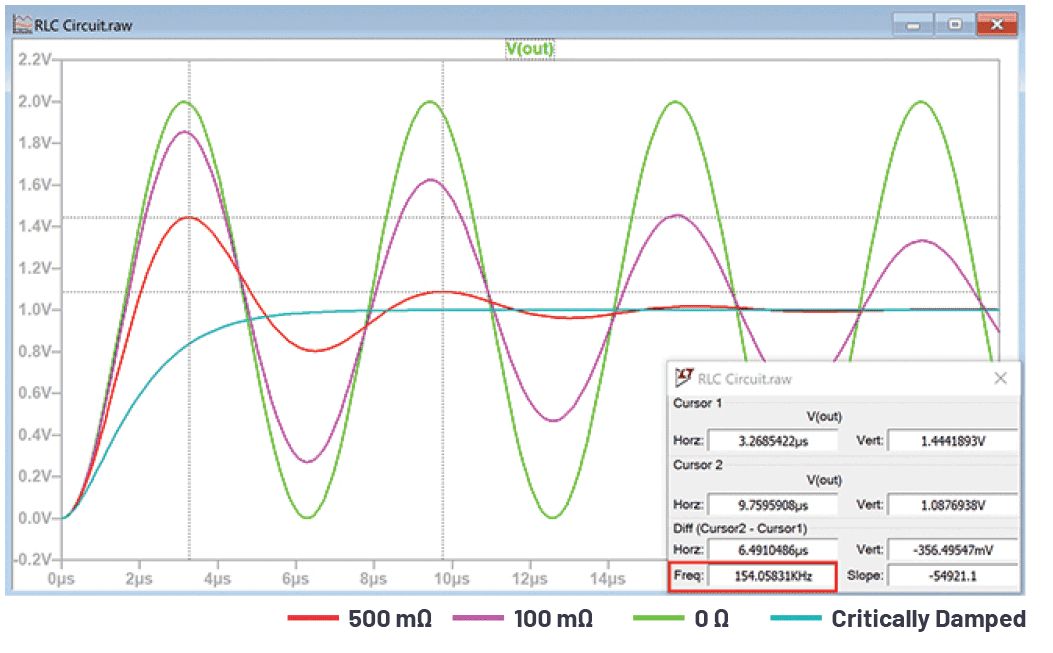Figure 4. The effect of damping on the amplitude and frequency of an RLC circuit.

The amplitude of the sine wave decays by e–ζωnt . With a damping coefficient of 0.25, a natural frequency, ωn, of 1 Mrads–1, and a damped natural frequency of 968246 rads–1, Equation 11 becomes

Using this formula, VOUT calculates to 1.44 V at 3.26 μs and 1.09 V at 9.75 μs, identical to the readings that can be seen in Figure 4.

Figure 4 clearly shows the effect of increasing the damping coefficient. Both the amplitude and the damped natural frequency reduce.

What happens if we keep increasing the damping coefficient?

We know that the damped natural frequency is given by

We can see that if the damping coefficient is increased to unity, the damped natural frequency reduces to zero. This is known as the point of critical damping, where all oscillation in the circuit stops. This can also be seen in Equation 11. Since the damped natural frequency, ωd, has reduced to zero, the sine term equates to zero, the cosine term equates to unity, and the expression simplifies to a first-order system—similar to a capacitor charging through a resistor.

This can be seen in the critically damped trace in Figure 4.

Unstable Systems

Since all circuits possess resistance, many electronic control circuits are inherently stable with poles lying in the left-half plane. However, from Equation 11, a negative damping coefficient causes an exponentially growing amplitude response, so poles lying in the right-half plane cause instability. With circuit simulation, it is easy to see the effect of a right-half plane pole by inserting a negative resistance. Figure 5 shows an RLC circuit, but where the resistance is negative.

In this circuit, the damping coefficient is –0.1. Figure 6 shows its response to a step input.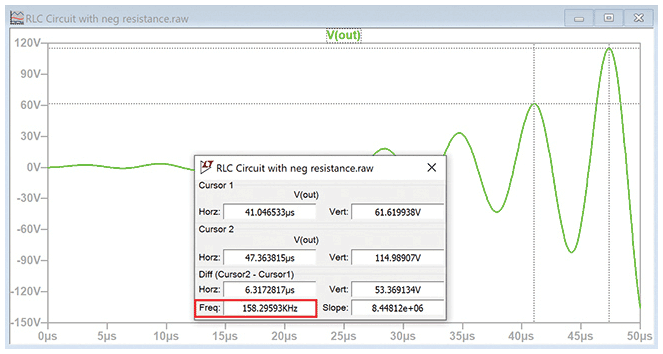Figure 6. The step response of a second-order system with negative damping.

The damped natural frequency is still dictated by

And, for a damping coefficient of –0.1, the actual frequency of oscillation is 994987 rads–1 (158.3 kHz).

Again, from Equation 11, the response of our circuit is dictated by

We can work out the amplitude response as the output grows: VOUT calculates to 61.62 V at 41.05 μs and to 114.99 V at 47.36 μs, which is identical to the readings shown in Figure 6.

Dominant Poles

Sometimes a system consists of many poles, making analysis complicated. However, if the poles are spaced sufficiently apart, the effect of one pole often dominates the others and the system can be simplified by ignoring the non-dominant poles.

The top half of Figure 7 shows two RLC circuits, each with identical L and C components; only the resistance has been changed. The circuit with the lower resistance has its pole closer to the imaginary axis in the s plane.Figure 7. The effect of dominant pole location on series and parallel circuits.

The bottom half of Figure 7 shows these two circuits in series. V(OUT3) has been replicated using a behavioral voltage source, B1, to avoid it being loaded with R4, L4, and C4 so we can see the true response of V(OUT3) × V(OUT4).Figure 8. The effect of a dominant pole on system response with two waveforms added or multiplied.

We can see their responses in Figure 8. Unsurprisingly, the circuit with the largest resistance has the largest damping coefficient, hence its oscillation decays the quickest, as seen in the plot of V(OUT2). However, we notice that when both outputs are either added (putting the circuits in parallel), or multiplied (putting the circuits in series), V(OUT1) dominates the response. Therefore, one way to simplify a complex system is to focus on the circuit that has poles closer to the jω axis, which tends to dominate the system response.

Systems with Poles in Both Left- and Right-Half Planes

We have considered systems with poles in either the left- or the right-half plane. What happens if the system has poles in both the left- and right-half planes? Which one wins the battle for stability and why?

Referring again to Equation 11, it is the exponent that determines if the system is stable. We can ignore the sinusoidal part of Equation 11 and just look at the exponents to see what happens if we combine a left-half pole with a right-half pole. Figure 9 shows a simple circuit to demonstrate this.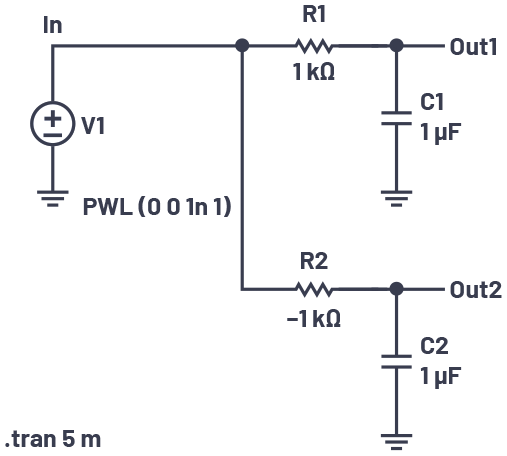Figure 9. A circuit with poles in both the left- and right-half planes.

The RC circuit at the top clearly has a left-half pole since its resistance is positive. The circuit at the bottom has a right-half pole. A mathematical derivation of this is shown in Appendix B.

The response of the circuit in Figure 9 is shown in Figure 10.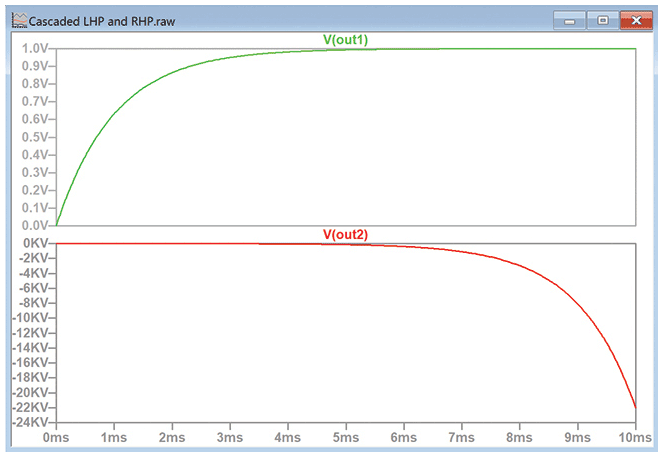Figure 10. The response to a step input of an RC circuit with positive and negative resistance.

The top waveform settles to a zero gradient after about 5 ms, matching the generally accepted rule that an RC circuit will settle in about five time constants. In contrast, V(OUT2) shows an ever-increasing gradient. It should now be apparent that if a circuit with a left-half plane pole is connected in series with a circuit with a right-half plane pole, then the complete circuit will be unstable since the right-half plane response continues to exponentially increase long after the left-half plane circuit has settled. So, for a circuit to be stable, all poles must lie in the left-half plane.

Conclusion

This article connects the theoretical models used in electronic control theory with the practical world of the electronics engineer. Control systems are stable if all poles lie in the left-half plane due to the resistance (or damping) present in the system. Practically demonstrating the response of a system with a right-half plane pole can prove problematic since it requires modeling a negative resistance. However, computer simulation comes to the rescue, enabling us to demonstrate stable and unstable circuits by simply changing the polarity of the resistance.

Likewise, Laplace transforms rarely manage to break out of the classroom, but here they have proved invaluable in proving how second-order electronic systems work.

Appendix A

Showing that

The Laplace transform of a unit step input is

The general transfer function of a second-order low-pass filter is given by

So the response of a second-order system stimulated by a unit step is given by

A standard partial fraction expansion exists and is given by

Replacing x with s gives

In A4 there is no s or s2 term in the numerator. Also, there is no a term in the denominator.

So Equation A6 can be rewritten as

So

To ensure both sides of Equation A8 have the same denominator, it can be rewritten as

As a check, the right hand side of Equation A9 can be compared with the right hand side of Equation A8:

We can now equate the numerators of Equation A9 to find A, B, and C:

Equating coefficients of s2:

0 = A + B

Equating coefficients of s1:

0 = A(2ζωn) + C

Equating coefficients of s0:

ωn2 = Aωn2

So A = 1, B = –1, C = –2ζωn

Therefore, from Equation A8

(note the change in sign because B and C are negative)

There are three transforms from the time domain (on the left) to the Laplace domain (on the right):

By completing the square, we can write Equation A12 as

Which equals

We now need to make the numerator equal to (s + ζωn) so it matches the first term in the denominator enabling us to use the Laplace identity:

So, by separating out ζωn into its own fraction, Equation A14 equals

(So a = –ζωn and b = ωn√(1 – ζ2))

We now need to make the numerator of the third term of Equation A17 equal to ωn√(1 – ζ2) so it matches the denominator and enables us to use the Laplace identity:

Dividing the third term of Equation A18 by ωn√(1 – ζ2), we can then put ωn√(1 – ζ2) on the numerator.

So the whole expression can be rewritten as

So a = –ζωn and b = ωn√(1 – ζ2)

Equation A19 can now be translated out of the Laplace domain as

The two wn’s cancel in the third term. Since the damped natural frequency, ωd, can be written as

Equation A20 can be simplified to read

Many textbooks state that the Equation A22 polynomial can also be written as

So we have a decaying exponential that is dependent on the damping coefficient and the undamped natural frequency and an oscillation dependent on the damped natural frequency.

Equation A23 can be entered into a spreadsheet and a graph plotted of the output in response to a step input.

Appendix B

Showing that

The Laplace transform of a unit step input is

The general transfer function of an RC circuit is given by

The denominator is equal to zero for negative values of s, therefore, for this circuit, the poles lie in the left-half plane, so the system is stable. If the resistance was negative, the poles would lie in the right-half plane and the system would be unstable.

From Equation B3, we can see that an RC circuit’s transfer function in response to a step input is given by

A standard partial fraction expansion exists and is given by

In this case, a = 0

so

Equating terms of s1 in the numerator gives

0 = ACR + B

Equating terms of s0 in the numerator gives

1 = A

So A = 1, B = –CR

So

There are two transforms from the time domain (on the left) to the Laplace domain (on the right):

So translating Equation B7 into the time domains means the RC responds according to

as expected.

Simulations were conducted in LTspice.